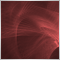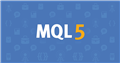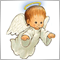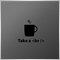# Converting double to integer function fails with some operations53

Good morning, I need to get the last digit of a price and "round it" according to some rules of my own, it's not just rounding it. To detect a price's last digit I'm multiplying the price for 100000 and %10 to obtain it. However, and even when a large part of operations I run this way works, there are some prices that make it fail. For example, I passed a price of 1.05964 and, when multiplied by 100000 it gives me 105963. It just makes no sense that the function works with some operations and fails with others. However, I'm aware of a Warning the compiler is prompting me: "possible loss of data due to type conversion". So, there might be a better option to do what I'm intending to. I'd be happy if someone could either help me with my current way of doing it or by providing me an alternative. Thanks!

```   double RoundPrice(string PassedTrend, double PassedPrice){

int Value= PassedPrice*100000;
int TailNumber = Value%10;
double BearishMinor = Point*(5-TailNumber);
double BearishMajor = Point*(10-TailNumber);
double BullishMinor = Point*TailNumber;
double BullishMajor = Point*(TailNumber-5);

if (PassedTrend == "Bearish" && TailNumber <=4){
double FinalPrice = PassedPrice + BearishMinor;
return(FinalPrice);
}if (PassedTrend == "Bearish" && TailNumber >= 5){
double FinalPrice = PassedPrice + BearishMajor;
return(FinalPrice);
}

if(PassedTrend == "Bullish" && TailNumber <=4){
double FinalPrice = PassedPrice - BullishMinor;
return(FinalPrice);
}if(PassedTrend == "Bullish" && TailNumber >=5){
double FinalPrice = PassedPrice - BullishMajor;
return(FinalPrice);
}else{
return(3.00000); // ErrorOP
}

}```4905

marclfp:

Good morning, I need to get the last digit of a price and "round it" according to some rules of my own, it's not just rounding it. To detect a price's last digit I'm multiplying the price for 100000 and %10 to obtain it. However, and even when a large part of operations I run this way works, there are some prices that make it fail. For example, I passed a price of 1.05964 and, when multiplied by 100000 it gives me 105963. It just makes no sense that the function works with some operations and fails with others. However, I'm aware of a Warning the compiler is prompting me: "possible loss of data due to type conversion". So, there might be a better option to do what I'm intending to. I'd be happy if someone could either help me with my current way of doing it or by providing me an alternative. Thanks!

How sure are you that the price was 1.05964  and not for example 1.059636 because that would explain it:

1059636 x 100000 would give 105963.6 but because you have cast to an integer you would get 105963

so for your solution to work you must make sure that the number you pass in has the correct number of decimal places, but in doing that you are going to be rounding up or down in the first instance and does that not conflict with your whole purpose?53

Paul Anscombe:

How sure are you that the price was 1.05964  and not for example 1.059636 because that would explain it:

1059636 x 100000 would give 105963.6 but because you have cast to an integer you would get 105963

so for your solution to work you must make sure that the number you pass in has the correct number of decimal places, but in doing that you are going to be rounding up or down in the first instance and does that not conflict with your whole purpose?

The price of 1.05964 is extracted directly from the High of a particular Bar. Since I've outputted that number in the form of an alert and it has given me exactly 1.05964 I've assumed that it has that number of digits. However, I don't know if the High of a Bar returns a value with more digits. If that were the case, that'd be the problem, as you've pointed out. Is it the case? Thanks for your answer.4905

marclfp:
The price of 1.05964 is extracted directly from the High of a particular Bar. Since I've outputted that number in the form of an alert and it has given me exactly 1.05964 I've assumed that it has that number of digits. However, I don't know if the High of a Bar returns a value with more digits. If that were the case, that'd be the problem, as you've pointed out. Is it the case? Thanks for your answer.

obviously you can use NormalizeDouble to ensure you have the correct number of decimal places to start with, but it may round your input number as mentioned above.

https://www.mql5.com/en/docs/convert/normalizedoubleDocumentation on MQL5: Conversion Functions / NormalizeDouble
• www.mql5.com
Calculated values of StopLoss, TakeProfit, and values of open prices for pending orders must be normalized with the accuracy, the value of which can be obtained by Digits(). Please note...53

Paul Anscombe:

obviously you can use NormalizeDouble to ensure you have the correct number of decimal places to start with, but it may round your input number as mentioned above.

https://www.mql5.com/en/docs/convert/normalizedouble

I've just tried it out, it didn't work. It seems the High of a Bar is already a 5 decimals number, so the problem shouldn't be there.3951

marclfp:

Good morning, I need to get the last digit of a price and "round it" according to some rules of my own, it's not just rounding it. To detect a price's last digit I'm multiplying the price for 100000 and %10 to obtain it. However, and even when a large part of operations I run this way works, there are some prices that make it fail. For example, I passed a price of 1.05964 and, when multiplied by 100000 it gives me 105963. It just makes no sense that the function works with some operations and fails with others. However, I'm aware of a Warning the compiler is prompting me: "possible loss of data due to type conversion". So, there might be a better option to do what I'm intending to. I'd be happy if someone could either help me with my current way of doing it or by providing me an alternative. Thanks!

This is a feature of floating-point numbers. Your number 1.05964 could be for example 1.059639987... If you assign a double number to integer number you don't round this double but just cut floating part.

Try this:

`int Value= int(round(PassedPrice*100000)+0.1);`53

Petr Nosek:

This is a feature of floating-point numbers. Your number 1.05964 could be for example 1.059639987... If you assign a double number to integer number you don't round this double but just cut floating part.

Try this:

Thanks a lot for the explanation and the code. It works and I've understood the bug. Have a great day!23248

1. NormalizeDouble returns a double. Using it before the multiply solved nothing.

2. Floating-point has an infinite number of decimals, it's your not understanding floating-point and that some numbers can't be represented exactly. (like 1/10.)
Double-precision floating-point format - Wikipedia, the free encyclopedia

If you want to see the correct number of digits, convert it to a string with the correct/wanted accuracy.
question about decima of marketinfo() - MQL4 programming forum 2016.05.18

3. `int Value= int(PassedPrice*100000 +0.5);`
4. Don't hard code numbers. Your code fails on JPY pairs. Use PassedPrice / _Point.3951

William Roeder:

`int Value= int(PassedPrice*100000 +0.5);`

In this example you are right because 0.5 is exactly represented as 0.5 and 100000 is exactly 100000. But if you don't hard code numbers (your good advice point 4.) and use division by _Point you can get in trouble (maybe) in some cases because _Point usually cannot be represented exactly.

BTW I don't know if all of (double) numbers without decimals can be represented exactly. If so then we can omit "+0.1" in my example.475

marclfp:

Good morning, I need to get the last digit of a price and "round it" according to some rules of my own, it's not just rounding it. To detect a price's last digit I'm multiplying the price for 100000 and %10 to obtain it. However, and even when a large part of operations I run this way works, there are some prices that make it fail. For example, I passed a price of 1.05964 and, when multiplied by 100000 it gives me 105963. It just makes no sense that the function works with some operations and fails with others. However, I'm aware of a Warning the compiler is prompting me: "possible loss of data due to type conversion". So, there might be a better option to do what I'm intending to. I'd be happy if someone could either help me with my current way of doing it or by providing me an alternative. Thanks!

```//METHOD 1
int GetLastDigits(double value,int digit,int howlong,int howmany)
{

if (howlong>digit)
{
Print("target reduced digit must be lesser than digit count");
return(0);
}

string originalvalue = string(value);
ushort separator = 46;
string resultsplit[];
int k = StringSplit(originalvalue,separator,resultsplit);
if (k<1)
{
Print("not a double/float");
return(0);
}

resultsplit = IsLastDigitZero(resultsplit,digit) ? resultsplit += "0" : resultsplit;
int length;

length = StringLen(resultsplit);

string returnsplit = (howlong<length) ? StringSubstr(resultsplit,0,howlong) : resultsplit;
length = StringLen(returnsplit);
string returndigit = StringSubstr(returnsplit,(length-howmany),howmany);

int returnvalue = int(returndigit);
return(returnvalue);
}

bool IsLastDigitZero(string decimals,int digit)
{
return(((int)StringLen(decimals)<digit) ? true : false);
}

void Test()
{
double price1 = 1.232834539; //9 digits
double price4  = 1.65470; //5 digits

Print("Last 1 digit price1 is ",IntegerToString(GetLastDigits(price1,5,5,1)));
Print("Last 1 digit price1 with digits reduced to 8 is ",IntegerToString(GetLastDigits(price1,8,9,1)));
Print("Last 1 digit price1 with digits reduced to 4 is ",IntegerToString(GetLastDigits(price1,5,4,1)));
Print("Last 1 digits price4 is ",IntegerToString(GetLastDigits(price4,5,5,1)));
}

```

this should help you get exactly the last digit..i usually use string if i want to work with double/float for high precision value based on my target digit..William is right..hope this helps...23248

Petr Nosek: use division by _Point you can get in trouble (maybe) in some cases because _Point usually cannot be represented exactly.

That is the purpose of the 0.5, rounding the answer to the nearest point (as int) in case of floating point round off.

Petr Nosek: BTW I don't know if all of (double) numbers without decimals can be represented exactly. If so then we can omit "+0.1" in my example.

Up to 15 significant digits.
Double-precision floating-point format - Wikipedia, the free encyclopedia

No, you can not. The 0.1 (yours) or 0.5 (mine) must be there to avoid floating point errors on conversion to integer. Multiplying by an exact value doesn't change the inexact price or the inexact result.